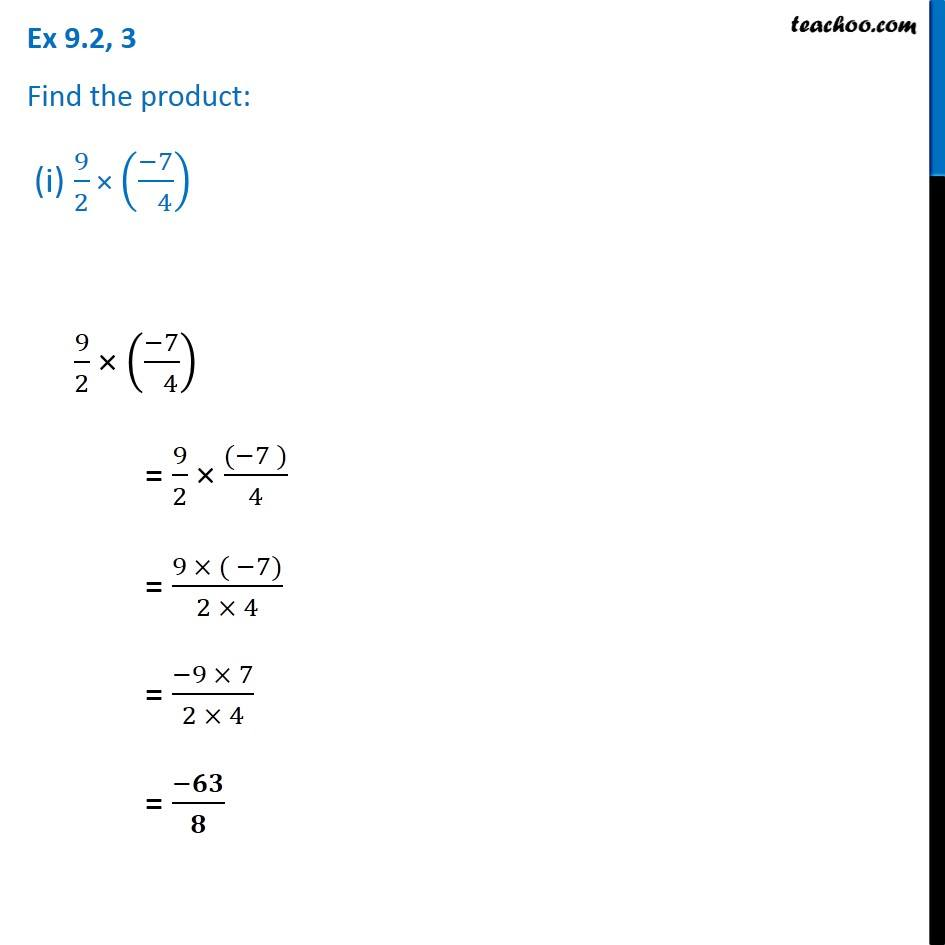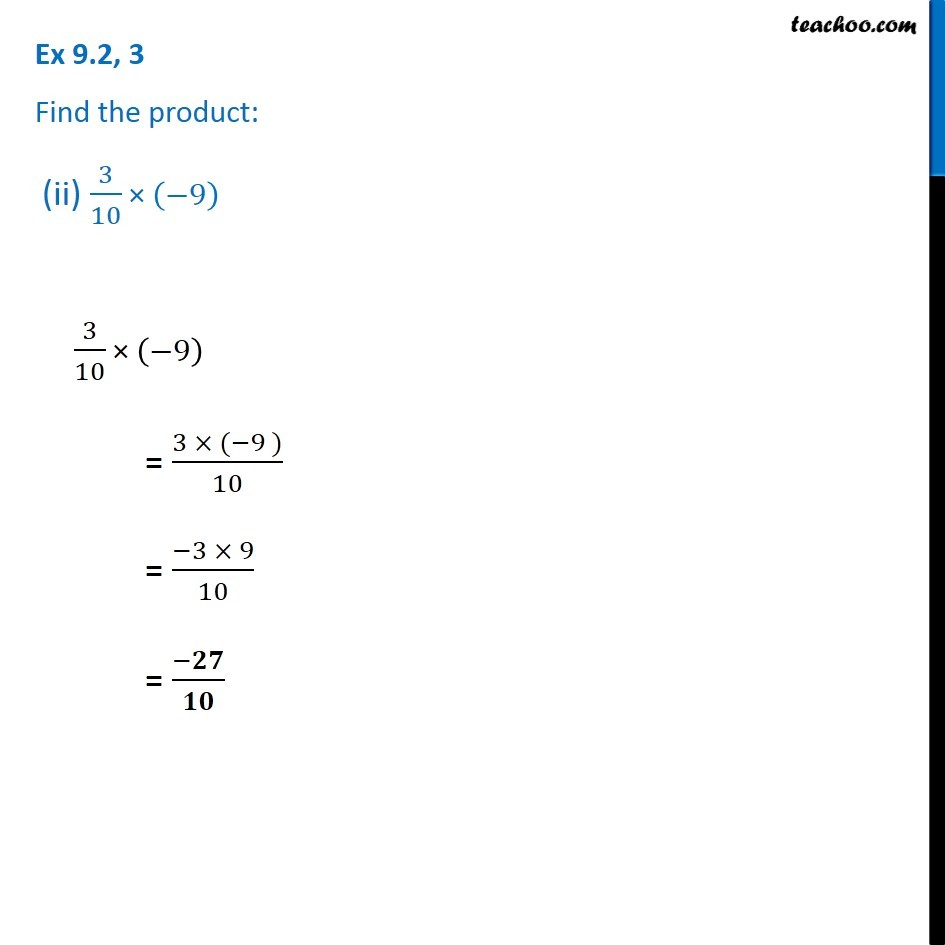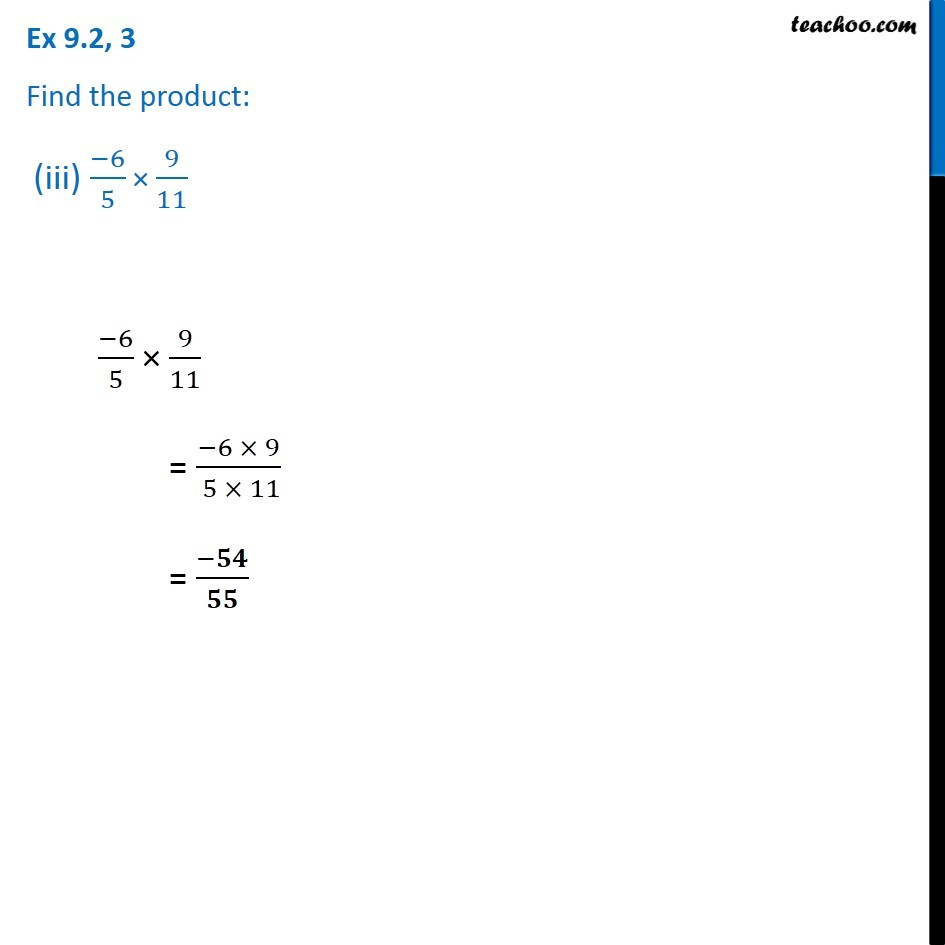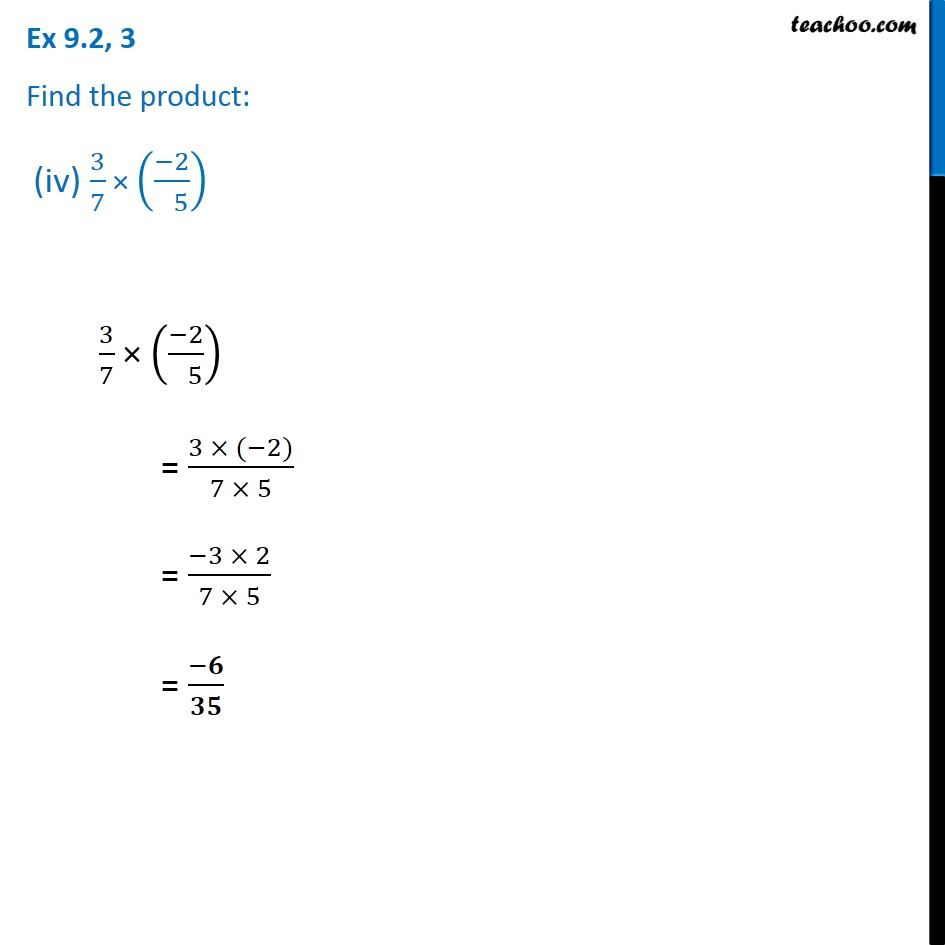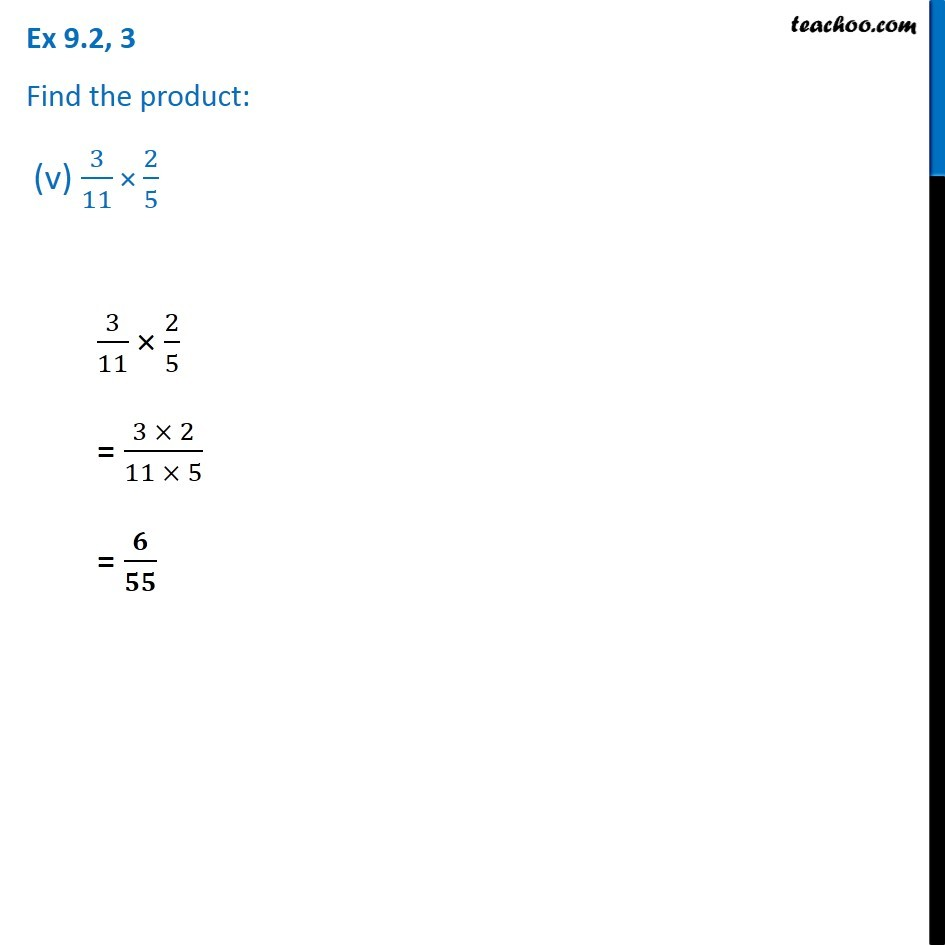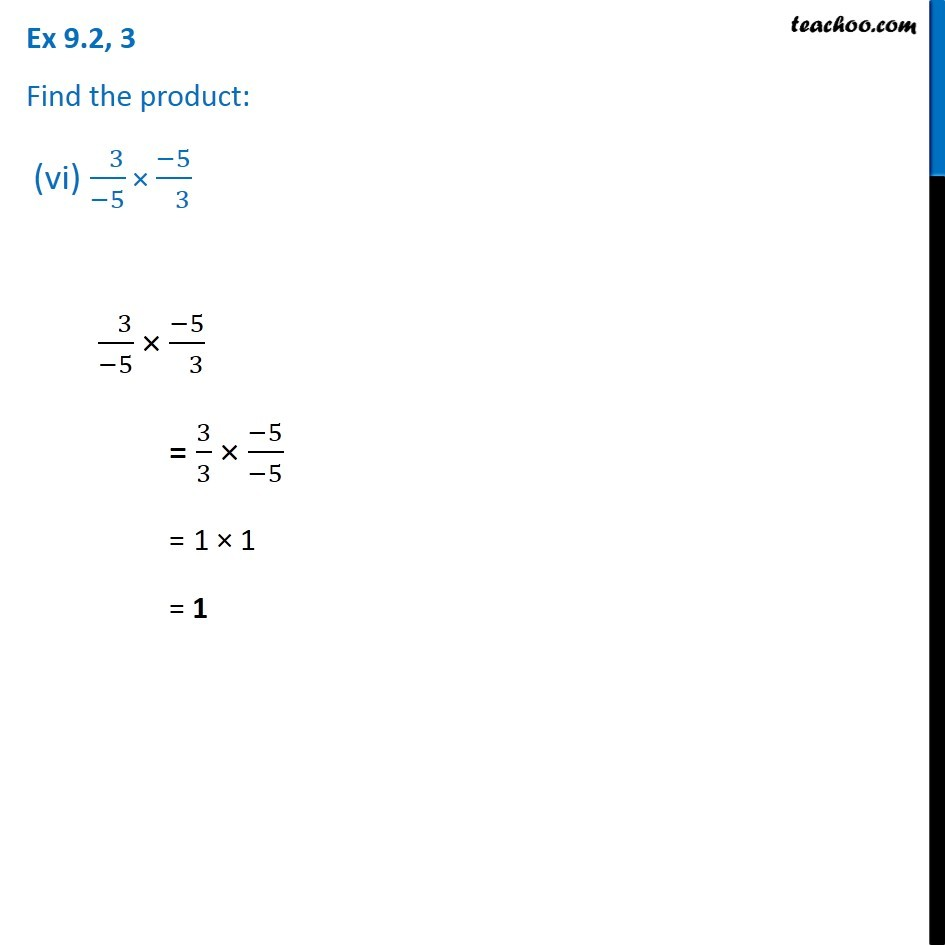1. Chapter 9 Class 7 Rational Numbers
2. Serial order wise
3. Ex 9.2

Transcript

Ex 9.2, 3 Find the product: (i) 9/2 × ((−7)/( 4)) 9/2 × ((−7)/( 4)) = 9/2 × ((−7 ))/4 = (9 × ( −7))/(2 × 4) = (−9 × 7)/(2 × 4) = (−𝟔𝟑)/𝟖 Ex 9.2, 3 Find the product: (ii) 3/10 × (−9) 3/10 × (−9) = (3 × (−9 ))/10 = (−3 × 9)/10 = (−𝟐𝟕)/𝟏𝟎 Ex 9.2, 3 Find the product: (iii) (−6)/5 × 9/11 (−6)/5 × 9/11 = (−6 × 9)/( 5 × 11) = (−𝟓𝟒)/𝟓𝟓 Ex 9.2, 3 Find the product: (iv) 3/7 × ((−2)/( 5)) 3/7 × ((−2)/( 5)) = (3 × (−2))/(7 × 5) = (−3 × 2)/(7 × 5) = (−𝟔)/𝟑𝟓 Ex 9.2, 3 Find the product: (v) 3/11 × 2/5 3/11 × 2/5 = (3 × 2)/(11 × 5) = 𝟔/𝟓𝟓 Ex 9.2, 3 Find the product: (vi) ( 3)/(−5) × (−5)/( 3) ( 3)/(−5) × (−5)/( 3) = 3/3 × (−5)/(−5) = 1 × 1 = 1

Ex 9.2